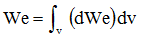# Explanation of results

## Conduction current and displacement current

The density of the conduction current is given by the post-processing parameter Jc, which corresponds to the following formula: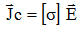The density of the total current (conduction current and displacement current) is given by the post-processing parameter J, which corresponds to the following formula: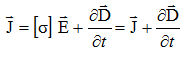## Powers: general overview

Contrary to Steady state AC Electric, in Transient Electric, the dielectric losses corresponding to tan (δ) of the material (corresponding also to the hysteresis losses of the D (E) law) are not considered in the computation.

The “losses” term corresponds to the ohmic losses and is equal to the “power” term.

Those two quantities are expressed by the following expressions:

• Instantaneous power: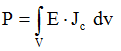• Losses: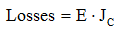## Electrostatic Energy

The density of electrostatic energy (mean value on one period) on one point is expressed by the following relation depending on D and E: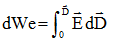Which can also be written, in linear, homogeneous and isotropic media: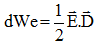The electrostatic energy (mean value on one period) in a volume region is equal to: